# Accumulation of errors

Jump to: navigation, search

in the numerical solution of algebraic equations

The overall effect of rounding-off at the various stages of a computation procedure on the accuracy of the computed solution to a system of algebraic equations. The most commonly employed technique for a priori estimation of the total effect of rounding-off errors in numerical methods of linear algebra is the scheme of inverse (or backward) analysis. Applied to the solution of linear algebraic equations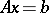(1)

the scheme of inverse analysis is as follows. On the assumption that some direct methodhas been used, the computed solutiondoes not satisfy (1), but it can be expressed as the exact solution of a perturbed system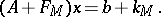(2)

The quality of the direct method is estimated in terms of the best a priori estimate that can be found for the norms of the matrix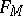and the vector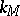. These "best"andare known as the equivalent perturbation matrix and vector, respectively, for the method.

If estimates forandare available, the error of the approximate solution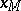can be estimated theoretically by the inequality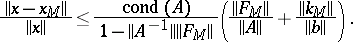(3)

Here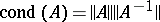is the condition number of the matrix, and the matrix norm in (3) is assumed to be subordinate to the vector norm.

In reality, an estimate for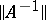is rarely known in advance, and the principal meaning of (2) is the possibility that it offers of comparing the merits of different methods. In the sequel some typical estimates for the matrixare presented.

For methods with orthogonal transformations and floating-point arithmetic (and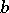in the system (1) are assumed to be real)(4)

In this estimate,is the relative precision of in the computer,is the Euclidean matrix norm, andis a function of type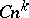, whereis the order of the system. The exact values of the constantsand the exponentsdepend on such details of the computation procedure as the rounding-off method, the use made of accumulation of inner products, etc. Most frequently,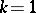or.

In Gauss-type methods, the right-hand side of the estimate (4) involves yet another factor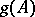, reflecting the possibility that the elements ofmay increase at intermediate steps of the method in comparison with their initial level (no such increase occurs in orthogonal methods). In order to reduceone resorts to various ways of pivoting, thus putting bounds on the increase of the matrix elements.

In the square-root method (or Cholesky method), which is commonly used when the matrixis positive definite, one has the sharper estimate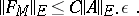There exist direct methods (the methods of Gordan, bordering and of conjugate gradients) for which a direct application of a scheme of inverse analysis does not yield effective estimates. In these cases different arguments are utilized to investigate the accumulation of errors (see ).